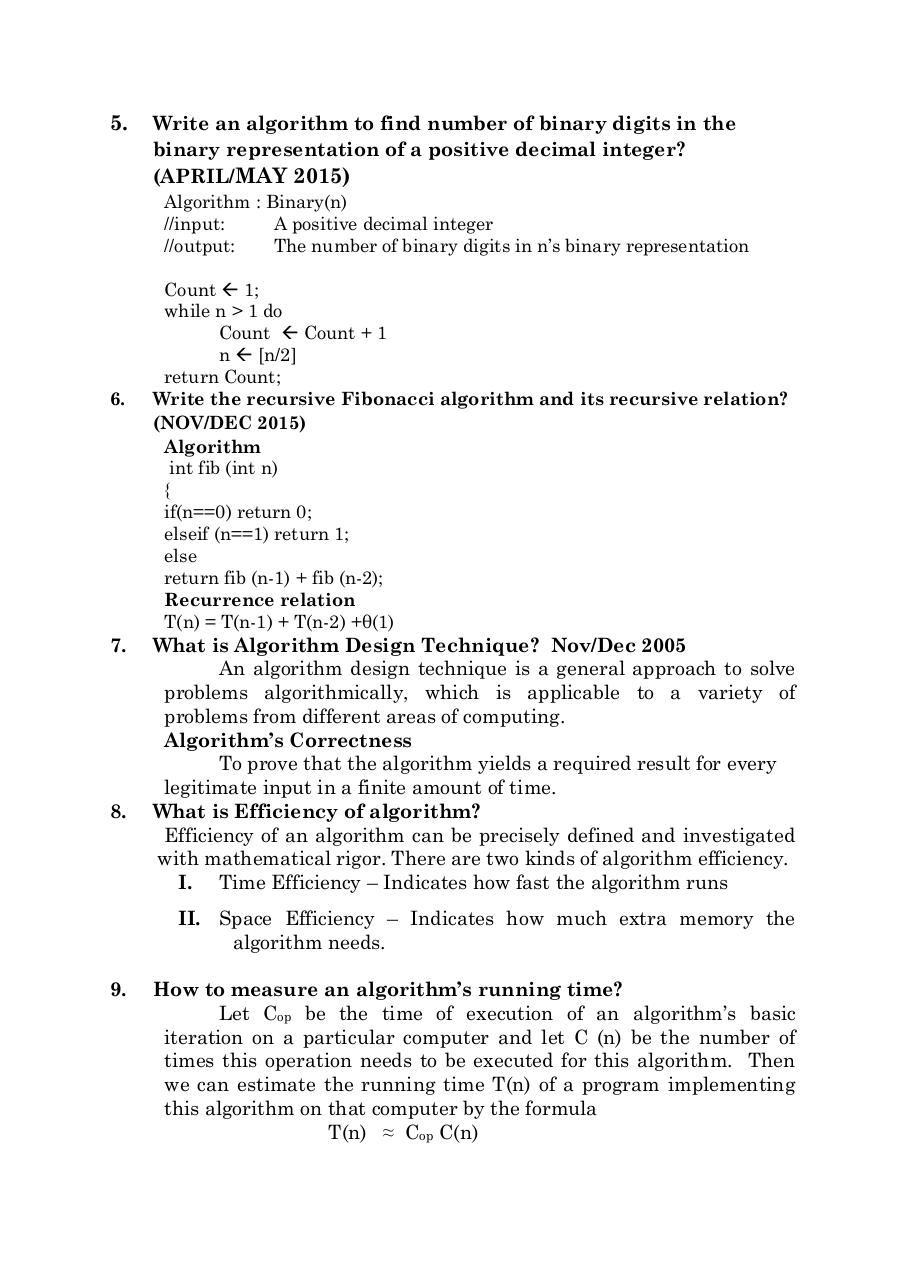# UNIT 1 QB.pdfPage 1 2 3 4 5 6 7 8

#### Text preview

5.

Write an algorithm to find number of binary digits in the
binary representation of a positive decimal integer?
(APRIL/MAY 2015)
Algorithm : Binary(n)
//input:
A positive decimal integer
//output:
The number of binary digits in n’s binary representation

6.

7.

8.

Count  1;
while n &gt; 1 do
Count  Count + 1
n  [n/2]
return Count;
Write the recursive Fibonacci algorithm and its recursive relation?
(NOV/DEC 2015)
Algorithm
int fib (int n)
{
if(n==0) return 0;
elseif (n==1) return 1;
else
return fib (n-1) + fib (n-2);
Recurrence relation
T(n) = T(n-1) + T(n-2) +θ(1)

What is Algorithm Design Technique? Nov/Dec 2005
An algorithm design technique is a general approach to solve
problems algorithmically, which is applicable to a variety of
problems from different areas of computing.
Algorithm’s Correctness
To prove that the algorithm yields a required result for every
legitimate input in a finite amount of time.
What is Efficiency of algorithm?
Efficiency of an algorithm can be precisely defined and investigated
with mathematical rigor. There are two kinds of algorithm efficiency.
I. Time Efficiency – Indicates how fast the algorithm runs
II. Space Efficiency – Indicates how much extra memory the
algorithm needs.

9.

How to measure an algorithm’s running time?
Let Cop be the time of execution of an algorithm’s basic
iteration on a particular computer and let C (n) be the number of
times this operation needs to be executed for this algorithm. Then
we can estimate the running time T(n) of a program implementing
this algorithm on that computer by the formula
T(n) ≈ Cop C(n)